# Torus

Jump to: navigation, search

Note that the term torus is often used for the more specific and restricted notion of 2-torus.

## Definition

A$n$-torus is defined as the product of$n$ copies of the circle, equipped with the product topology. In other words, it is the space$S^1 \times S^1 \times \dots \times S^1$ with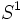$S^1$ written$n$ times.

Cases of special interest are$n = 1$ (where we get the circle) and$n = 2$ (where we get the 2-torus).

The$n$-torus is sometimes denoted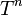$T^n$, a convention we follow on this page.

## Algebraic topology

### Homology

Further information: homology of torus

The homology (with integer coefficients)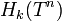$H_k(T^n)$ is a free abelian group of rank$\binom{n}{k}$ for$0 \le k \le n$, and is the zero group for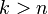$k > n$ (note that under one of the interpretations of binomial coefficient, we do not need to make a separate case for$k > n$ because$\binom{n}{k}$ is defined to be zero for$k > n$).

More generally, the homology with coefficients in a module$M$ over a commutative unital ring$R$ is$H^k(T^n;M) \cong M^{\binom{n}{k}}$.

### Cohomology

Further information: cohomology of torus

The cohomology groups are isomorphic to the respective homology groups, both with integer coefficients and with coefficients in an arbitrary module.

The cohomology ring with coefficients in a commutative unital ring$R$ is the alternating algebra in$n$ variables over$R$.

### Homotopy

Further information: homotopy of torus

Each torus is an aspherical space as well as a path-connected space, so its only nontrivial homotopy group is the fundamental group, which is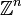$\mathbb{Z}^n$. In other words: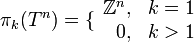$\pi_k(T^n) = \lbrace\begin{array}{rl} \mathbb{Z}^n, & k = 1 \\ 0, & k > 1 \\\end{array}$